# AP Chemistry Practice Test 32

### Test Information13 questions19 minutes

1. Which of the reactions below will not be thermodynamically favored at all temperatures?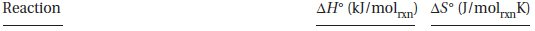2. Consider the following possible mechanism for this gas phase reaction above: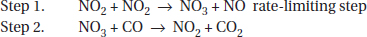Which of the following statements is not true?

3. An experiment was performed to determine the moles of carbon dioxide gas formed (collected over water) when an acid reacts with limestone. To do this, a piece of dry limestone was weighed. Then carbon dioxide was collected until the limestone disappeared. The atmospheric pressure was measured at 0.965 atm. The temperature was 295 K. The volume of CO2 was measured to the nearest mL and corrected for the vapor pressure of water. The student continuously got low results from what was expected. Can you suggest why?

4.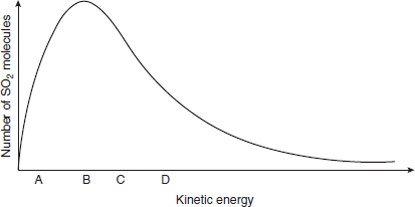Choose the description of the point on the distribution curve that is the most plausible.

5. Your supervisor asks you to determine the enthalpy of a certain chemical reaction. Which would you do?

6. A 25 g sample of a solid was heated to 100 °C and then quickly transferred to an insulated container holding 100 g of water at 26 °C. The temperature of the mixture rose to reach a final temperature of 37 °C. Which of the following can be concluded?

7.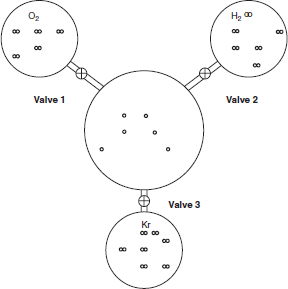Three 1-liter flasks are connected to a 3-liter flask by valves as shown in the diagram above. The 3-liter flask has the relative number of helium atoms as indicated. At the start, the entire system is at 585K. The first flask contains oxygen; the second contains hydrogen, and the third contains nitrogen. The pressure of hydrogen is 3.00 atm. The number of gas molecules is proportional to their representations in the flasks. If the valves are all opened, what will be the pressure in the system? Assume the connections have negligible volume.

8.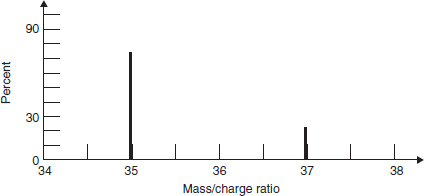A mass spectrum of a naturally occurring sample of an element is shown above. What is the element?

`Questions 9-13 refer to the following information.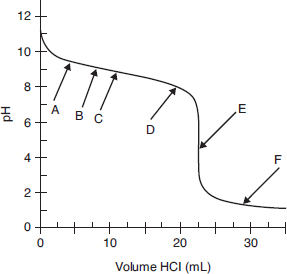The above graph shows a titration curve of a weak base titrated with a strong acid. The pH was measured with a pH meter after small volumes of 0.200 M HNO3 were added to 50.0 mL of a weak base. Data from that experiment are shown in that graph.`

9. Which of the following describes the base that is being titrated?

10. At what point on this curve does the solution display the least buffering capacity?

11. At what point on the curve does the equation MacidVacid = MbaseVbase ?

12. If the student uses a pH indicator that changes color from pH 8 to 6, which statement best characterizes the expected observations?

13. Which arrow points to the place where the slope (ΔpH/Δvol) of the titration curve is the smallest?